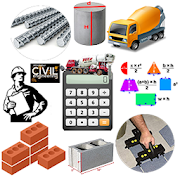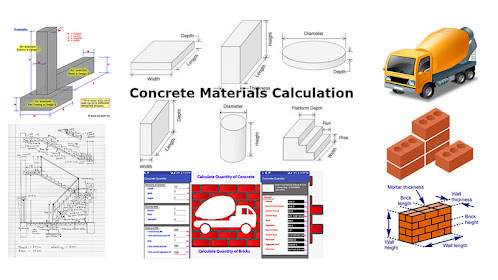# Construction Calculator (Concrete, Steel, Bricks)Everyone
744
Construction calculator is a Calculating platform for all the civil Engineers and Construction Workers around the world.
Calculate material for construction and other construction calculations.
Calculate Concrete, Bricks, Steel, Blocks, Tiles and Area etc. All in one.

The Construction Calculator for your Android smartphone is built with the same features and functions, and the same core engine that powers the industry-standard advanced construction Calculators from Aink Studio.

Construction Calculator could help You to calculate allotment of materials for house building, inches to feet, construction materials, construction estimator, construction cost estimating or maintenance. because Construction Calculator is a materials estimating for building construction. Gives You opportunity to check your contractor for overspend and moment provide you necessary information.

Save time with solutions for Contractors, Builders, Carpenters, Architects, Engineers, Trades persons and Construction estimator.

Construction Calculator is a simple tool to calculate volume of footings, slab, square column, bar column, strip foundation, bricks wall, concrete blocks wall and steps. It also calculate total cost and quantity of concrete needed for construction of strip foundation, bricks, concrete blocks, area, finishing tiles, reinforcement steel, footings, slab, square column, bar column, and steps.

Construction Calculator features :
Bricks Calculator
Steel Calculator
Concrete Calculator
Blocks Calculator
Best building cost estimator
Paint Calculator
Tiles Calculator
Plaster Calculator
building cost calculator
building materials
construction estimator
concrete estimator
Area Calculator
Slab Concrete Calculator
Slab Steel Calculator
Cube Concrete Calculator
Round Concrete Calculator
Concrete Column Calculator
Square Area Calculator
RCC Slab Calculator
Rectangle Area Calculator
Triangle Area Calculator
Circle Area Calculator
Parallelogram Calculator.
Calculate Concrete Quantity of Cement, Sand, Aggregate and Cost With Full Details.
Calculate Bricks Quantity of Cement, Sand, number of Bricks and Cost With Full Details.

1) Calculates volume and total cost for different types of strip footings, slab, square column, round column, bricks wall, blocks wall and steps.

2) Calculates quantity of concrete needed for construction of footings, slab, square column, round column, and steps.

3) Calculates quantity of bricks needed for construction of bricks wall.
4) Calculates quantity of concrete blocks needed for construction of blocks wall.
5) Calculates quantity of Tiles needed for construction of room or bathroom floor and wall.

6) Calculates quantity of weight steel needed for construction of RCC slab, RCC Column and RCC Beam.

7) Calculates Area needed for construction.
8) Calculates concrete mix – Amount of cement bags, sand, gravel and total concrete for given volume.

9) Calculates Wall Bricks – Amount of bricks and Cement bags for given required Materials.

10) Calculates Wall Blocks – Amount of blocks and Cement bags for given required Materials.

11) Calculates Room or Bathroom tiles – Amount of tiles and Cement bags for given required Materials.

12) Calculates steel weight– Amount of steel weight for given required weight.

- Calculates quantity of bricks needed to build a wall.
- Calculates quantity of Column Concrete needed to build a Column.
- Calculates quantity of Slab Concrete needed to build a Slab.
- Calculates weight and total cost of a steel bar.
- You can set metric or imperial measurement units.
- Calculate amount of bricks for building different size walls.
- Calculate amount of gas-concrete, foam-concrete or another block for building different size walls. Estimate rough cost of materials.
- It is free, fast, simple and easy to use.

This app specially developed for Civil engineer, Construction estimator, Builder and Construction Worker but any one can use it.
Collapse

Review Policy
4.6
744 total
5
4
3
2
1

## What's New

New Features added Concrete, Bricks, Steel, Building Estimator,
Tiles, Blocks, RCC Concrete and Area With Full Details
Easy to Use
Collapse

Updated
October 28, 2019
Size
4.8M
Installs
100,000+
Current Version
1.73
Requires Android
4.2 and up
Content Rating
Everyone
Permissions
Offered By
Aink Studio
Developer
Postal Code 28350, kpk, Pakistan